cancel
Showing results for
Did you mean:## Use conditional formatting to mark the same aggregated value in the same row in the matrix

Scenario:

When using different columns as filters, judge whether the column aggregate values of the results of each row are equal, and use the background color as a label.

Sample data:

Raw Data in Power QueryVisual in Report View: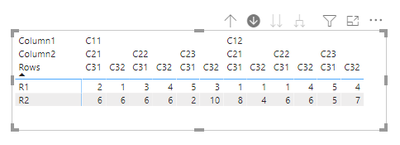Operations:

We can use a DAX measure in conditional formatting to mark rows.

Before writing a measure, we need to consider two points:

• How to compare whether each aggregated value in the same row is the same?
• How to ensure the comparison is at the same level?

``````Measure=
VAR t123 =
SUMMARIZE (
FILTER ( ALL ( 'Table' ), [Rows] = MAX ( 'Table'[Rows] ) ),
[Rows],
[Column1],
[Column2],
[Column3],
"Result123", SUM ( 'Table'[Values] )
)
VAR t12 =
SUMMARIZE (
FILTER ( ALL ( 'Table' ), [Rows] = MAX ( 'Table'[Rows] ) ),
[Rows],
[Column1],
[Column2],
"Result12", SUM ( 'Table'[Values] )
)
VAR t1 =
SUMMARIZE (
FILTER ( ALL ( 'Table' ), [Rows] = MAX ( 'Table'[Rows] ) ),
[Rows],
[Column1],
"Result1", SUM ( 'Table'[Values] )
)
VAR t2 =
SUMMARIZE (
FILTER ( ALL ( 'Table' ), [Rows] = MAX ( 'Table'[Rows] ) ),
[Rows],
[Column2],
"Result2", SUM ( 'Table'[Values] )
)
VAR t3 =
SUMMARIZE (
FILTER ( ALL ( 'Table' ), [Rows] = MAX ( 'Table'[Rows] ) ),
[Rows],
[Column3],
"Result3", SUM ( 'Table'[Values] )
)
RETURN
IF (
ISINSCOPE ( 'Table'[Column3] ),
IF (
NOT ( ISINSCOPE ( 'Table'[Column2] ) ),
IF (
COUNTROWS ( FILTER ( t3, [Result3] = SUM ( 'Table'[Values] ) ) )
= COUNTROWS ( t3 ),
1,
0
),
IF (
COUNTROWS ( FILTER ( t123, [Result123] = SUM ( 'Table'[Values] ) ) )
= COUNTROWS ( t123 ),
1,
0
)
),
IF (
ISINSCOPE ( 'Table'[Column2] ),
IF (
NOT ( ISINSCOPE ( 'Table'[Column1] ) ),
IF (
COUNTROWS ( FILTER ( t2, [Result2] = SUM ( 'Table'[Values] ) ) )
= COUNTROWS ( t2 ),
1,
0
),
IF (
COUNTROWS ( FILTER ( t12, [Result12] = SUM ( 'Table'[Values] ) ) )
= COUNTROWS ( t12 ),
1,
0
)
),
IF (
ISINSCOPE ( 'Table'[Column1] ),
IF (
COUNTROWS ( FILTER ( t1, [Result1] = SUM ( 'Table'[Values] ) ) )
= COUNTROWS ( t1 ),
1,
0
)
)
)
) ``````

The idea is that in each hierarchy of level, calculate the total count in each row and make the total count equal to the current value, if they are equal, it means that the values in this row are equal.

In my formula, the var function appears many times in my formula. By using a variable, you can get the same outcome, but in a more readable way. In addition, the result of the expression is stored in the variable upon declaration. It doesn’t have to recalculate each time it is used, as it does when variables are not in use. This can improve the measure's performance.

Use summarize function to construct a virtual table to get the context you want without being affected by the context in visual.

Use isinscope function to restrict calculations in the same hierarchy.

Use countrow function to calculate number of rows in the context.

Generally, the colors defined by conditional formats are selected in the format, but if you use the measure, you can select ‘format by rules’ and specify what color to use for eligible rules.

Like this: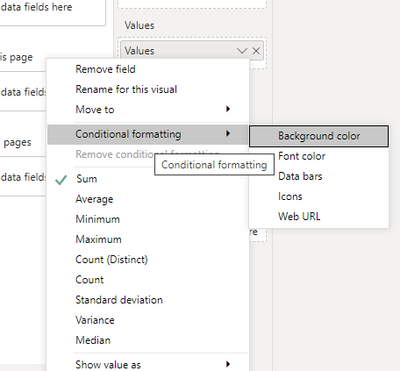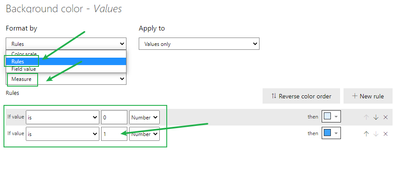Here’s the final result:When using Column1 as the filter condition: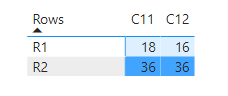The aggregate values of each column in R1 are not equal, so they are light in color, while the columns in R2 are not.

When using Column3 as the filter condition:The aggregate values of each column in R1 are not equal, so they are light in color, while the columns in R2 are not.

Similarly, when you drill down: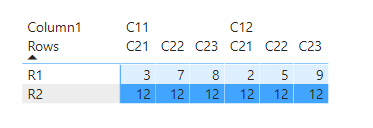Summary

If you want to analyze data in a matrix with hierarchies and need to quickly and clearly see rows of the same value, you can use 'isinscope' function to customize a measure for marking, and then use the 'Rules' function in the conditional format to display it.

Please refer to the attachment for details.

Author:  Janey Guo

Reviewer: Ula Huang, Kerry Wang

Top Kudoed Posts
Latest Articles
Archives
Polls
What is your favorite Power BI feature release for September 2023?